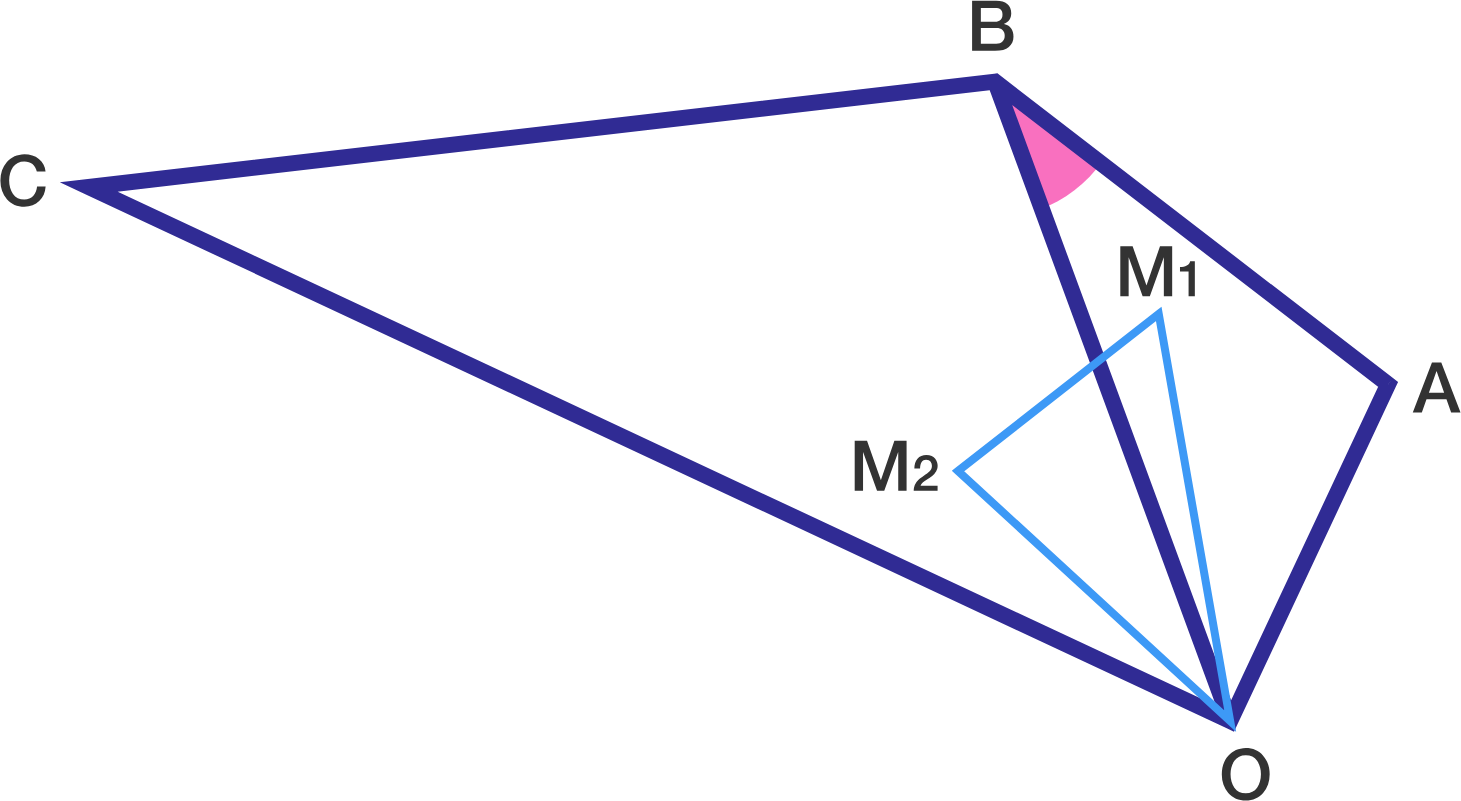# Similar Triangles and Centroids

Geometry Level 3Triangle $AOB$ and triangle $BOC$ are directly similar (they have the same orientation). Let the centroids of these two triangles be $M_1$ and $M_2$, respectively.

If triangle $M_1OM_2$ is right, and $M_1M_2=AB$, then the sum of all possible values of $\cos^2(\angle ABO)$ can be expressed as $\dfrac{p}{q}$ for positive coprime integers $p,q$.

Find $p+q$.

##### Note: We do not know which angle of $\triangle M_1 O M_2$ is the right angle.
×

Problem Loading...

Note Loading...

Set Loading...